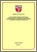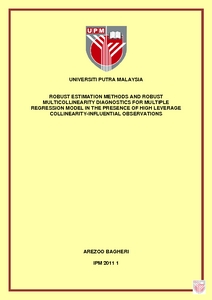# Robust Estimation Methods and Robust Multicollinearity Diagnostics for Multiple Regression Model in the Presence of High Leverage Collinearity-Influential Observations

## Citation

Bagheri, Arezoo (2011) Robust Estimation Methods and Robust Multicollinearity Diagnostics for Multiple Regression Model in the Presence of High Leverage Collinearity-Influential Observations. PhD thesis, Universiti Putra Malaysia.

## Abstract

The presence of outliers and multicollinearity are inevitable in real data sets and they have an unduly effect on the parameter estimation of multiple linear regression models. It is now evident that outliers in the X-direction or high leverage points are another source of multicollinearity. These leverage points may induce or hide near-linear dependency of explanatory variables in a data set. We call these leverages, high leverage collinearity-influential observations either enhancing or reducing multicollinearity. By proposing High Leverage Collinearity-Influential Measure, denoted as HLCIM, we study several criteria such as sample size and magnitude, percentage, and position of high leverage points which cause these leverages to change the multicollinearity pattern of collinear and non-collinear data sets. The Ordinary Least Squares (OLS) estimates are heavily influenced by the presence of high leverage collinearity-influential observations. To rectify this problem, two new groups of robust regression methods are proposed. The Diagnostic Robust Generalized Potentials (DRGP) based on Minimum Volume Ellipsoid (MVE) is incorporated with different types of robust methods such as L1, LTS, M, and MM in the establishment of the first proposed group of robust methods. The new proposed methods are called GM-DRGP-L1, GM-DRGP-LTS (or Modified GM-estimator1(MGM1)), M-DRGP, MM-DRGP, and DRGP-MM. The second group of the proposed robust methods is formulated by modifying the existing Generalized M-estimator which is called as GM6. Two new GM-estimators which we call the Modified GM-estimator 2 and the Modified GM-estimator 3, denoted as MGM2 and MGM3, respectively are developed. Some indicators are employed to assess the performance of several existing robust methods and the new proposed methods. The results for real data set and Monte Carlo simulation study reveal that our proposed MGM3 outperforms the OLS and some of the existing robust methods. The classical multicollinearity diagnostic methods may not be suitable to diagnose correctly the existence of multicollinearity in the presence high leverage collinearity-influential observations. To remedy this problem, two different approaches are proposed in the establishment of robust multicollinearity diagnostic methods. In the first approach, we propose robust variance inflation factors, namely the RVIF(MM) and the RVIF(MGM3). The later is based on the proposed robust coefficient determination of MGM3. In the second approach, the diagnostic robust methods are proposed, specifically the Robust Condition Number (RCN), Robust Variance Inflation Factors (RVIF) and Robust Variance Decomposition Properties (RVDP) which are based on Minimum Covariance Determinant (MCD). The findings of this study suggest that the developed robust multicollnearity diagnostic methods are able to identify the source of multicollinearity in non-collinear data sets in the presence of high leverage collinearity-enhancing observations. On the other hand, for collinear data sets, in the presence of high leverage collinearity-reducing observations, the developed robust multicollinearity diagnostic methods are able to diagnose the multicollinearity pattern of the data set, correctly. This thesis also addresses the problems of identifying multiple high leverage collinearity- influential observations in a data set. Since, the existing collinearity-influential measures fail to identify multiple collinearity-influential observations in a data set, a new High Leverage Collinearity-Influential Measure based on DRGP, denoted as HLCIM(DRGP) is proposed. The results of the study signify that this new diagnostic measure surpasses the existing measures. Furthermore, some non-parametric cutoff points for the proposed and some existing collinearity-influential measures are suggested in this thesis. High leverage points may be considered as good or bad leverage point which depend on their residuals values. Unfortunately, researchers do not consider good leverage points to be problematic. However, these points may be collinearity-influential observations and need more attention. Regression diagnostic plots are one of the easiest and efficient tools for virtualizing the influential observations in a data set. Unfortunately, there is no existing plot in the literatures that identifies high leverage collinearity-influential observations. Finally, in this regard, we proposed three diagnostic plots specifically the SR(LMS)-DRGP, the DRGP-HLCIM, and the SR(LMS)-HLCIM. These new proposed diagnostic plots serve as powerful tools in separating outliers in the y-direction and the X-direction and able to identify any high leverage point which is collinearity-influential observationPreview
PDF
IPM_2011_1.pdfView Item